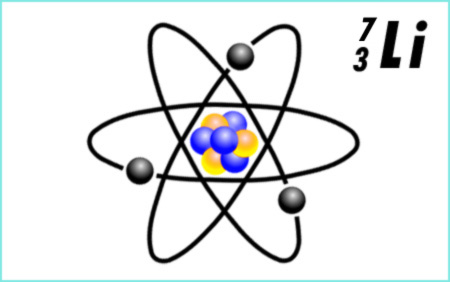Knowledge database: Stoichiometry: Mass and atomic number Mass number (A) is a number that shows the total number of particles, i.e. protons and neutrons, which the nucleus of an element contains. It is indicated by superscript numbers. Atomic number (Z) is the number of protons contained in the nucleus of a certain element. It is indicated by subscript numbers. In the periodic table of elements, the ordinal number of each element is equal to the atomic number. Thanks to this number, we know how many protons an atom of a specific element has in its nucleus, and how many electrons there are in its electron cloud. The above described mass number is therefore equal to the sum of the atomic number (number of protons) and the number of neutrons in the nucleus, which can vary. Atoms of the same element with different numbers of neutrons are called isotopes. Because of a different number of neutrons, their relative atomic masses are also different. More about the relative atomic mass can be found in the next chapter.On the picture above, a simplified visualization of a lithium atom is shown, with the atomic number 3, which means that this atom has 3 protons in its nucleus (the yellow particles), and that it has 3 electrons in its electron cloud. The mass number is 7, which means that besides the 3 protons, there must also be 4 neutrons, which are visible as blue particles on the picture.